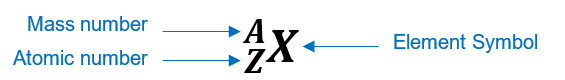# Problem: Identify the following elements. 83209X

###### FREE Expert Solution

We have to identify the given element: 83209X.

Recall that the symbol of an element is written is this way:Atomic number (Z):

• represents the identity of the element

• reveals the number of protons

• in a neutral element:

number of protons = number of electrons

Mass number (A)

• reveals the number of protons and neutrons

mass number = number of protons + number of neutrons###### Problem Details

Identify the following elements.

83209X